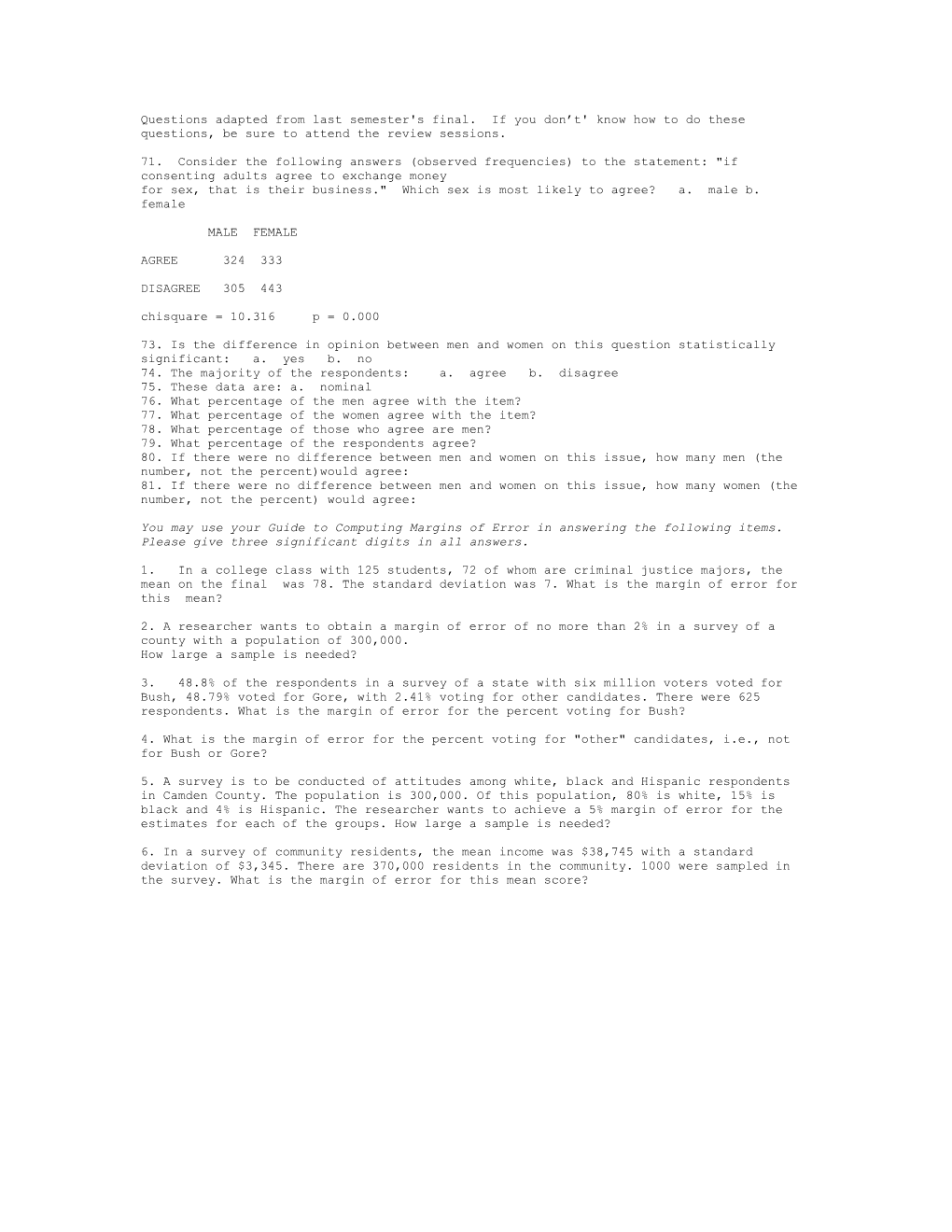# Questions Adapted from Last Semester's FinalQuestions adapted from last semester's final. If you don’t' know how to do these questions, be sure to attend the review sessions.

71. Consider the following answers (observed frequencies) to the statement: "if consenting adults agree to exchange money

for sex, that is their business." Which sex is most likely to agree? a. male b. female

MALE FEMALE

AGREE 324 333

DISAGREE 305 443

chisquare = 10.316 p = 0.000

73. Is the difference in opinion between men and women on this question statistically significant: a. yes b. no

74. The majority of the respondents: a. agree b. disagree

75. These data are: a. nominal

76. What percentage of the men agree with the item?

77. What percentage of the women agree with the item?

78. What percentage of those who agree are men?

79. What percentage of the respondents agree?

80. If there were no difference between men and women on this issue, how many men (the number, not the percent)would agree:

81. If there were no difference between men and women on this issue, how many women (the number, not the percent) would agree:

You may use your Guide to Computing Margins of Error in answering the following items. Please give three significant digits in all answers.

1. In a college class with 125 students, 72 of whom are criminal justice majors, the mean on the final was 78. The standard deviation was 7. What is the margin of error for this mean?

2. A researcher wants to obtain a margin of error of no more than 2% in a survey of a county with a population of 300,000.

How large a sample is needed?

3. 48.8% of the respondents in a survey of a state with six million voters voted for Bush, 48.79% voted for Gore, with 2.41% voting for other candidates. There were 625 respondents. What is the margin of error for the percent voting for Bush?

4. What is the margin of error for the percent voting for "other" candidates, i.e., not for Bush or Gore?

5. A survey is to be conducted of attitudes among white, black and Hispanic respondents in Camden County. The population is 300,000. Of this population, 80% is white, 15% is black and 4% is Hispanic. The researcher wants to achieve a 5% margin of error for the estimates for each of the groups. How large a sample is needed?

6. In a survey of community residents, the mean income was \$38,745 with a standard deviation of \$3,345. There are 370,000 residents in the community. 1000 were sampled in the survey. What is the margin of error for this mean score?

7. A study of Rutgers Camden Sociology Department graduates showed that the mean annual salary was \$55,000 with a standard deviation of \$4500. Threehundred graduates were sampled. What is the margin of error for this statistic?

Consider the data in the scattergram.

1. Is the relationship statistically significant?
1. Is the relationship positive or negative
1. Which is the independent variable? The dependent variable?
1. If an individual was 65 inches tall, how much would we predict that he or she weighed?
1. If an individual was 72 inches tall, how much would we predict that he or she would weigh?

A regression analysis with three independent variables gave the following results. Weight was measured in pounds, height in inches and gender was a dummy variable defined as (male=0, female = 1)

Analysis of Variance

Dependent Variable: weight

N: 22 Missing: 0

Multiple R-Square = 0.749 Y-Intercept = -74.697

Standard error of the estimate = 18.372

LISTWISE deletion (1-tailed test) Significance Levels: **=.01, *=.05

SourceSum of SquaresDFMean SquareFProb.

REGRESSION18144.32936048.11017.9190.000

RESIDUAL6075.48918337.527

TOTAL24219.81821

Unstand.bStand.BetaStd.Err.bt

Height3.1970.3861.544 2.071

age1.3980.2020.869 1.610

sex-34.006-0.49312.525-2.715 *

1. How much would we expect a 27 year old female who was 60 inches tall to weigh?

9. How much would we expect a 20 year old meal who was 72 inches tall to weigh?